## How to Calculate and Solve for Reaction: Lift Falls Freely | Motion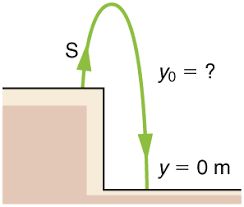The image above represents reaction: lift falls freely.

To compute for reaction: lift falls freely, two essential parameters are needed and these parameters are mass (m) and acceleration due to gravity (g).

The formula for calculating reaction: lift falls freely:

R = mg

Where;

R = Reaction
m = Mass
g = acceleration due to gravity

Let’s solve an example;
Find the reaction when the mass is 30 and acceleration due to gravity is 9.8.

This implies that;

m = Mass = 30
g = acceleration due to gravity = 9.8

R = mg
R = 30 x 9.8
R = 294

Therefore, the reaction is 294 N.

Calculating the Mass when the Reaction and the Acceleration due to Gravity is Given.

m = R / g

Where;

m = Mass
R = Reaction
g = acceleration due to gravity

Let’s solve an example;
Find the mass when the reaction is 40 and the acceleration due to gravity is 9.8

This implies that;

R = Reaction = 40
g = acceleration due to gravity = 9.8

m = R / g
m = 40 / 9.8
m = 40 / 9.8
m = 4.08

Therefore, the mass is 4.08.

## How to Calculate and Solve for Reaction: Lift Moves Down | Motion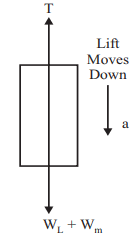The image above represents reaction: lift moves down.

To compute for reaction: lift moves down, three essential parameters are needed and these parameters are mass (m), acceleration (a) and acceleration due to gravity (g).

The formula for calculating reaction: lift moves down:

R = m(g – a)

Where;

R = Reaction
m = Mass
g = acceleration due to gravity
a = Acceleration

Let’s solve an example;
Find the reaction when the mass is 28, acceleration is 9 and acceleration due to gravity is 9.8.

This implies that;

m = Mass = 28
g = acceleration due to gravity = 9.8
a = Acceleration = 9

R = m(g – a)
R = 28(9.8 – 9)
R = 28(0.80)
R = 22.4

Therefore, the reaction is 22.4 N.

Calculating the Mass when the Reaction, the Acceleration and the Acceleration due to Gravity is Given.

m = R / g – a

Where;

m = Mass
R = Reaction
g = acceleration due to gravity
a = Acceleration

Let’s solve an example;
Find the mass when the reaction is 42, the acceleration is 8 and the acceleration due to gravity is 9.8

This implies that;

R = Reaction = 42
g = acceleration due to gravity = 9.8
a = Acceleration = 8

m = R / g – a
m = 42 / 9.8 + 8
m = 42 / 17.8
m = 2.359

Therefore, the mass is 2.359.

## How to Calculate and Solve for Reaction: Lift Moves Up | Motion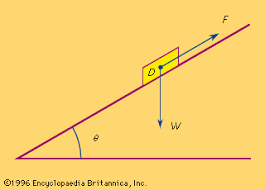The image above represents reaction: lift moves up.

To compute for reaction: lift moves up, three essential parameters are needed and these parameters are mass (m), acceleration (a) and acceleration due to gravity (g).

The formula for calculating reaction: lift moves up:

R = m(a + g)

Where;

R = Reaction
m = Mass
g = acceleration due to gravity
a = Acceleration

Let’s solve an example;
Find the reaction when the mass is 18, acceleration is 21 and acceleration due to gravity is 9.8.

This implies that;

m = Mass = 18
g = acceleration due to gravity = 21
a = Acceleration = 9.8

R = m(a + g)
R = 18(21 + 9.8)
R = 18(30.8)
R = 554.4

Therefore, the reaction is 554.4 N.

Calculating the Mass when the Reaction, the Acceleration and the Acceleration due to Gravity is Given.

m = R / a + g

Where;

m = Mass
R = Reaction
g = acceleration due to gravity
a = Acceleration

Let’s solve an example;
Find the mass when the reaction is 42, the acceleration is 21 and the acceleration due to gravity is 9.8

This implies that;

R = Reaction = 42
g = acceleration due to gravity = 9.8
a = Acceleration = 21

m = R / a + g
m = 42 / 21 + 9.8
m = 42 / 30.8
m = 1.36

Therefore, the mass is 1.36.

## How to Calculate and Solve for Recoil Velocity | Motion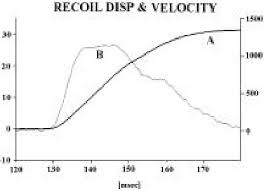The image above represents recoil velocity.

To compute for recoil velocity, three essential parameters are needed and these parameters are M, V and m.

The formula for calculating recoil velocity:

v = (-(M) x V) / m

Let’s solve an example;
Find the recoil velocity when M is 24, V is 10 and m is 6.

This implies that;

M = 24
V = 10
m = 6

v = (-(24) x 10) / 6
v = (-240) / 6
v = -40

Therefore, the recoil velocity is -40.

## How to Calculate and Solve for Force | Motion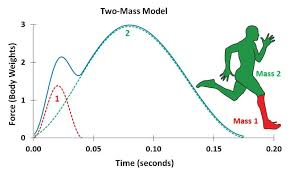The image above represents force.

To compute for force, two essential parameters are needed and these parameters are mass (m) and acceleration (a).

The formula for calculating force:

F = ma

Where;

F = Force
m = Mass
a = Acceleration

Let’s solve an example;
Find the force when the mass is 12 and the acceleration is 26?

This implies that;

m = Mass = 12
a = Acceleration = 26

F = ma
F = 12 x 26
F = 312

Therefore, the force is 312 N.

Calculating for Mass when the Force and the Acceleration is Given.

m = F / a

Where;

m = Mass
F = Force
a = Acceleration

Let’s solve an example;
Find the mass when the force is 100 and the acceleration is 20.

This implies that;

F = Force = 100
a = Acceleration = 20

m = F / a
m = 100 / 20
m = 5

Therefore, the mass is 5.

## How to Calculate and Solve for Impulse | Motion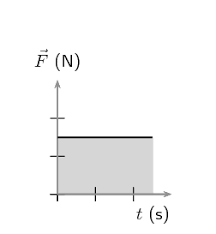The image above represents impulse.

To compute for impulse, two essential parameters are needed and these parameters are force (f) and time (t).

The formula for calculating impulse:

I = Ft

Where;

I = Impulse
F = Force
t = Time

Let’s solve an example;
Given that the force is 28 and the time is 14. Find the impulse?

This implies that;

F = Force = 28
t = Time = 14

I = Ft
I = 28 x 14
I = 392

Therefore, the impulse is 392 Ns.

Calculating the Force when the Impulse and the Time is Given.

F = I / t

Where;

F = Force
I = Impulse
t = Time

Let’s solve an example;
Given that the impulse is 60 and the time is 10. Find the force?

This implies that;

I = Impulse = 60
t = Time = 10

F = I / t
F = 60 / 10
F = 6

Therefore, the force is 6 N.

## How to Calculate and Solve for Linear Momentum | Motion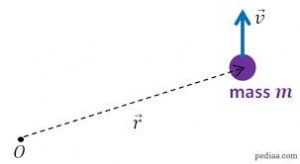The image above represents linear momentum.

To compute for linear momentum, two essential parameters are needed and these parameters are mass (m) and velocity (v).

The formula for calculating linear momentum:

p = mv

Where;

p = Momentum
m = Mass
v = Velocity

Let’s solve an example;
Find the momentum when the mass is 8 and the velocity is 12.

This implies that;

m = Mass = 8
v = Velocity = 12

p = mv
p = 8 x 12
p = 96

Therefore, the momentum is 96 Kgm/s.

Calculating the Mass when the Momentum and the Velocity is Given.

m = p / v

Where;

m = Mass
p = Momentum
v = Velocity

Let’s solve an example;
Find the mass when the momentum is 40 and the velocity is 18.

This implies that;

p = Momentum = 40
v = Velocity = 18

m = p / v
m = 40 / 18
m = 2.22

Therefore, the mass is 2.22 kg.

## How to Calculate and Solve for Velocity | Motion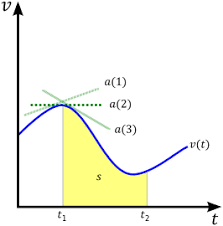The image above represents velocity.

To compute for velocity, three essential parameters are needed and these parameters are angular velocity (ω), amplitude (A) and displacement (y).

The formula for calculating velocity:

v = ω√(A² – y²)

Where;

v = Velocity
ω = Angular Velocity
A = Amplitude
y = Displacement

Let’s solve an example;
Find the velocity when the angular velocity is 7, amplitude is 10 and displacement is 13.

This implies that;

ω = Angular Velocity = 7
A = Amplitude = 10
y = Displacement = 13

v = ω√(A² – y²)
v = 7 x √(10² – 13²)
v = 7 x √(100 – 169)
v = 7 x √(-69)

i signifies a complex number = √(-1).

v = 7 x √(69) x √(-1)
v = 7 x √(69)i
v = 7 x 8.30i
v = 7(8.30i)

Therefore, the velocity is 7(8.30i) m/s.

Calculating the Angular Velocity when the Velocity, the Amplitude and the Displacement is Given.

ω = v / √(A2 – y2)

Where;

ω = Angular Velocity
v = Velocity
A = Amplitude
y = Displacement

Let’s solve an example;
Find the angular velocity when the velocity is 28, the amplitude is 14 and the displacement is 10.

This implies that;

v = Velocity = 28
A = Amplitude = 14
y = Displacement = 10

ω = v / √(A2 – y2)
ω = 28 / √(142 – 102)
ω = 28 / √(196 – 100)
ω = 28 / √(96)
ω = 28 / 9.79
ω = 2.86

Therefore, the angular velocity is 2.86.

## How to Calculate and Solve for Angular Velocity | Motion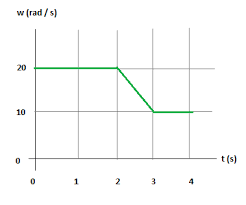The image above represents angular velocity.

To compute for angular velocity, two essential parameters are needed and these parameters are mass (m) and elastic constant (k).

The formula for calculating angular velocity:

ω = √(k / m)

Where;

ω = Angular Velocity
k = Elastic Constant
m = Mass

Let’s solve an example;
Find the angular velocity when the mass is 11 and the elastic constant is 28.

This implies that;

k = Elastic Constant = 28
jm = Mass = 11

ω = √(k / m)
ω = √(28 / 11)
ω = √(2.54)
ω = 1.59

Therefore, the angular velocity is 1.59 rad/s.

Calculating the Elastic Constant when the Angular Velocity and the Mass is Given.

k = ω2m

Where;

k = Elastic Constant
ω = Angular Velocity
m = Mass

Let’s solve an example;
Find the elastic constant when the angular velocity is 12 and the mass is 8.

This implies that;

ω = Angular Velocity = 12
m = Mass = 8

k = ω2m
k = 1228
k = 144 x 8
k = 1152

Therefore, the elastic constant is 1152.

## How to Calculate and Solve for Final Velocity | Motion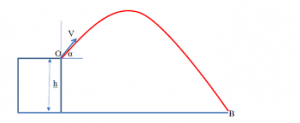The image above represents final velocity.

To compute for final velocity, three essential parameters are needed and these parameters are initial velocity (u), acceleration (a) and time (t).

The formula for calculating final velocity:

v = u + at

Where;

v = Final Velocity
u = Initial Velocity
a = Acceleration
t = Time

Let’s solve an example;
Find the Final velocity when the initial velocity is 12, acceleration is 9 and the time is 24.

This implies that;

u = Initial Velocity = 12
a = Acceleration = 9
t = Time = 24

v = u + at
v = 12 + (9 x 24)
v = 12 + 216
v = 228

Therefore, the final velocity is 228 m/s.

Calculating the Initial Velocity when the Final Velocity, the Acceleration and the Time is Given.

u = v – at

Where;

u = Initial Velocity
v = Final Velocity
a = Acceleration
t = Time

Let’s solve an example;
Find the Initial Velocity when the Final Velocity is 32, the acceleration is 12 and the time is 2.

This implies that;

v = Final Velocity = 32
a = Acceleration = 12
t = Time = 2

u = v – at
u = 32 – (12 x 2)
u = 32 – 24
u = 8

Therefore, the initial velocity is 8 m/s.